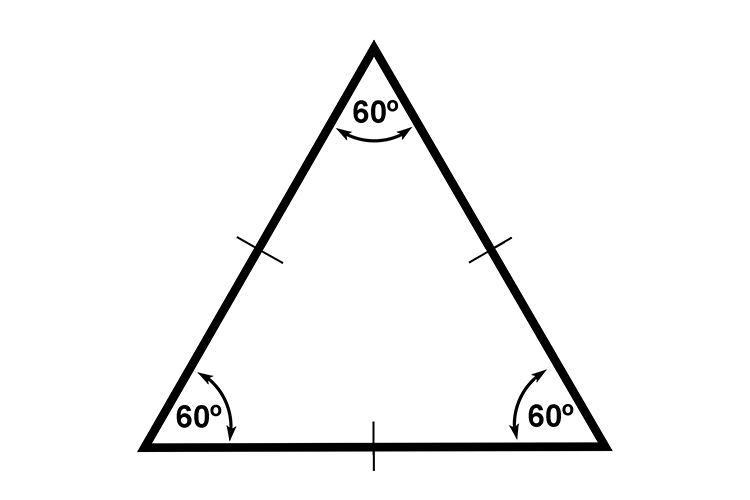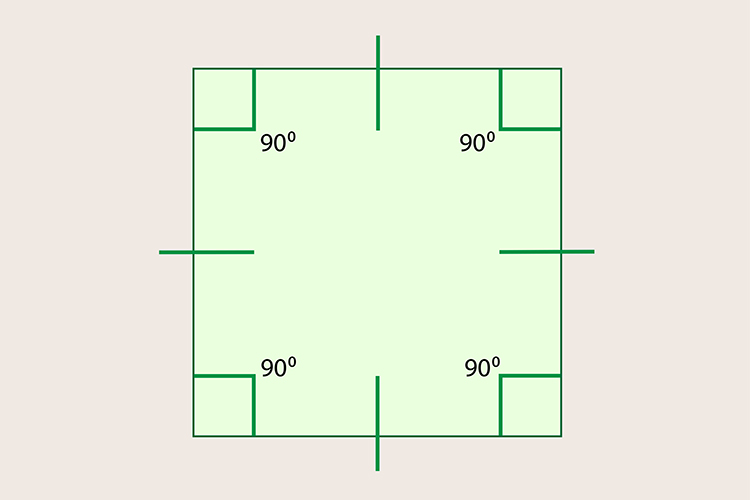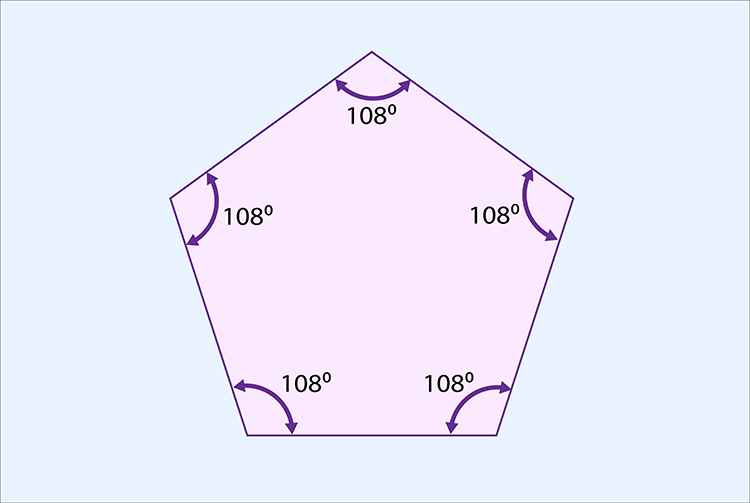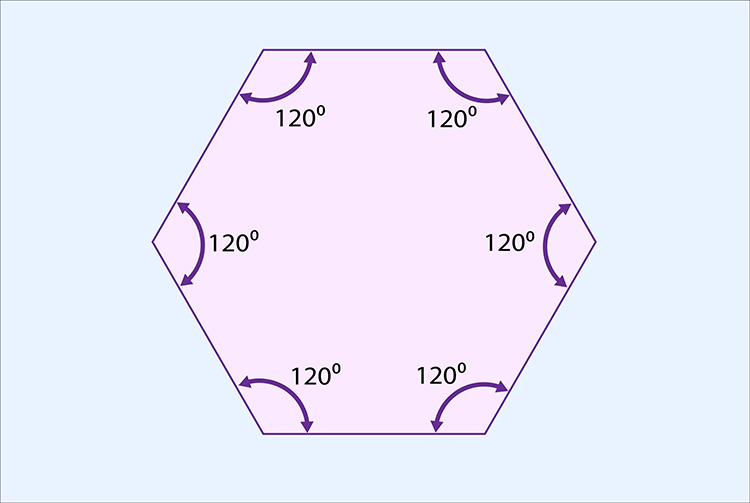# How do you know?If you can divide 360^@ exactly by an inside angle of a regular shape and get a whole number it can form a tessellation pattern.

## Triangle360/60=6

(6 is a whole number)

6 therefore this triangle can form a tessellation.

## Square360/90=4

(4 is a whole number)

A square can form a tessellation.

## Pentagon360/108=3.33

(3.33 is NOT a whole number)360/120=3